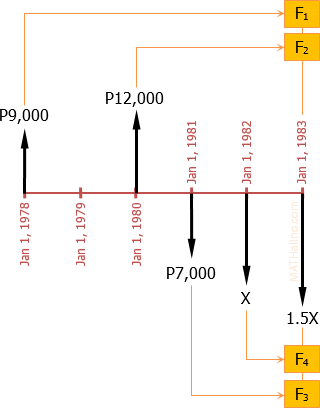# interest and compound: amount of loan payment

5 posts / 0 new
Sydney Salesinterest and compound: amount of loan payment

jones corporation borrowed P 9000 from Brown Corporation on Jan. 1, 1978 and P12000 on Jan.1,1980.Jones Corporation made a partial payment of P 7000 on Jan. 1, 1981. it was agreed that was the balance of the loan woild be amortizes by two payments one of Jan. 1, 1982 and the other on Jan.1, 1983. The second being 50% larger than the first. If the interest rate is 12%. What is the amount of each payment.

Sydney Salespasagot po.

Jhun VertThe activity of cash can be represented by the cash flow diagram below. The idea of the solution is to equate all the payments to all the debts in a common time. What we did below is we equate all cash activities in Jan. 1, 1983.$F = P(1 + i)^n$

$F_1 = 9,000(1 + 0.12)^5 = \text{P}15,861.08$

$F_2 = 12,000(1 + 0.12)^3 = \text{P}16,859.14$

$F_3 = 7,000(1 + 0.12)^2 = \text{P}8,780.80$

$F_4 = X(1 + 0.12) = 1.12X$

$1.5X + F_3 + F_4 = F_1 + F_2$

$1.5X + 8,780.80 + 1.12X = 15,861.08 + 16,859.14$

$2.62X = 23,939.42$

$X = \text{P}9,137.18$

$1.5X = \text{P}13,705.77$

Sydney Salessir thanks po.

KHERL BAGON MAR... (guest)

SALAMAT MGA LODICAKES

• Mathematics inside the configured delimiters is rendered by MathJax. The default math delimiters are $$...$$ and $...$ for displayed mathematics, and $...$ and $...$ for in-line mathematics.